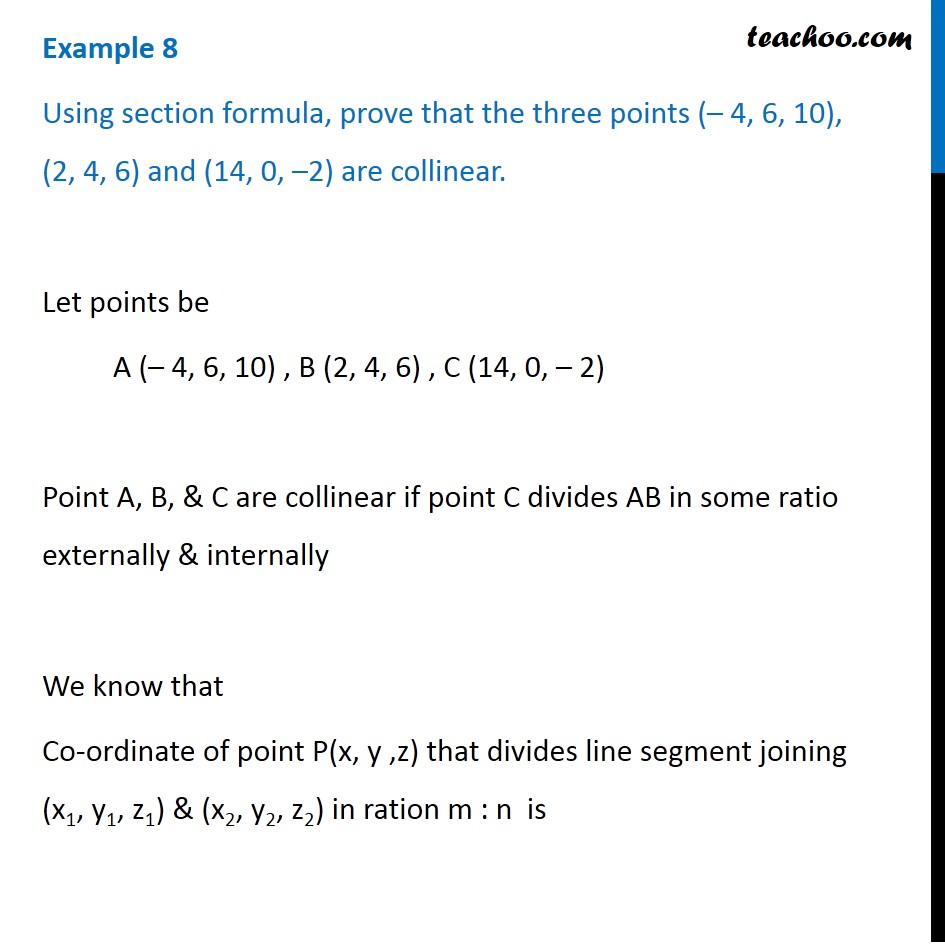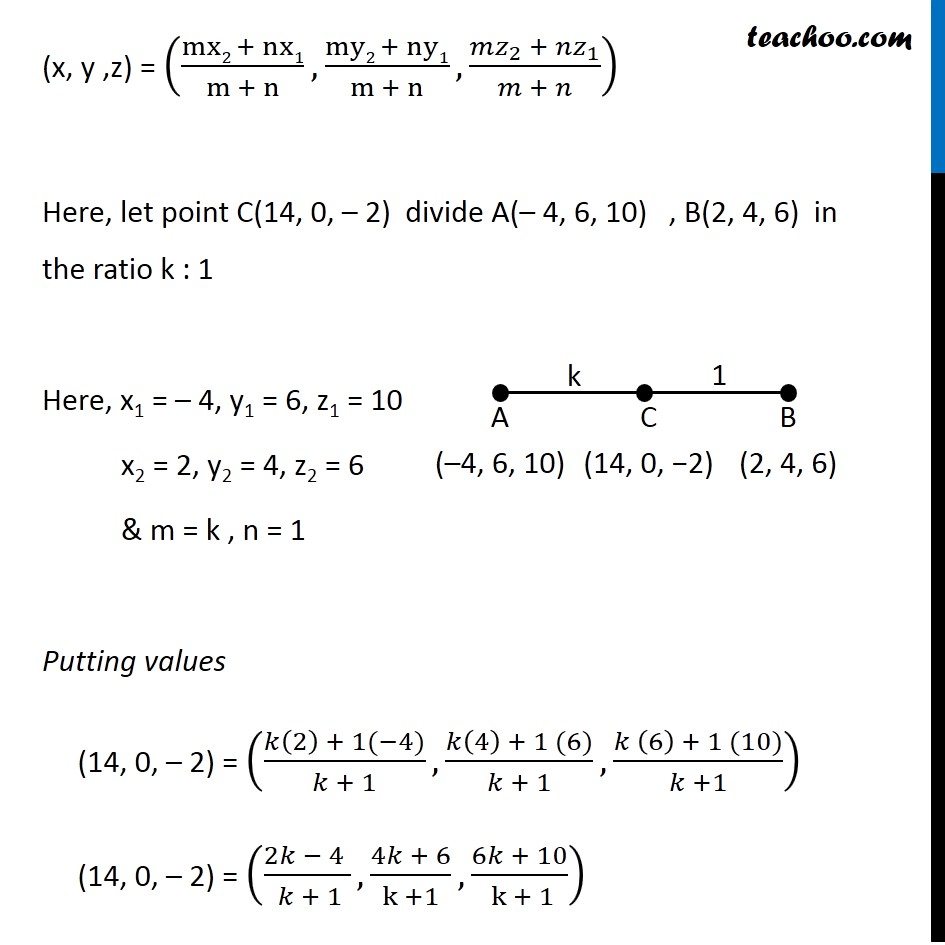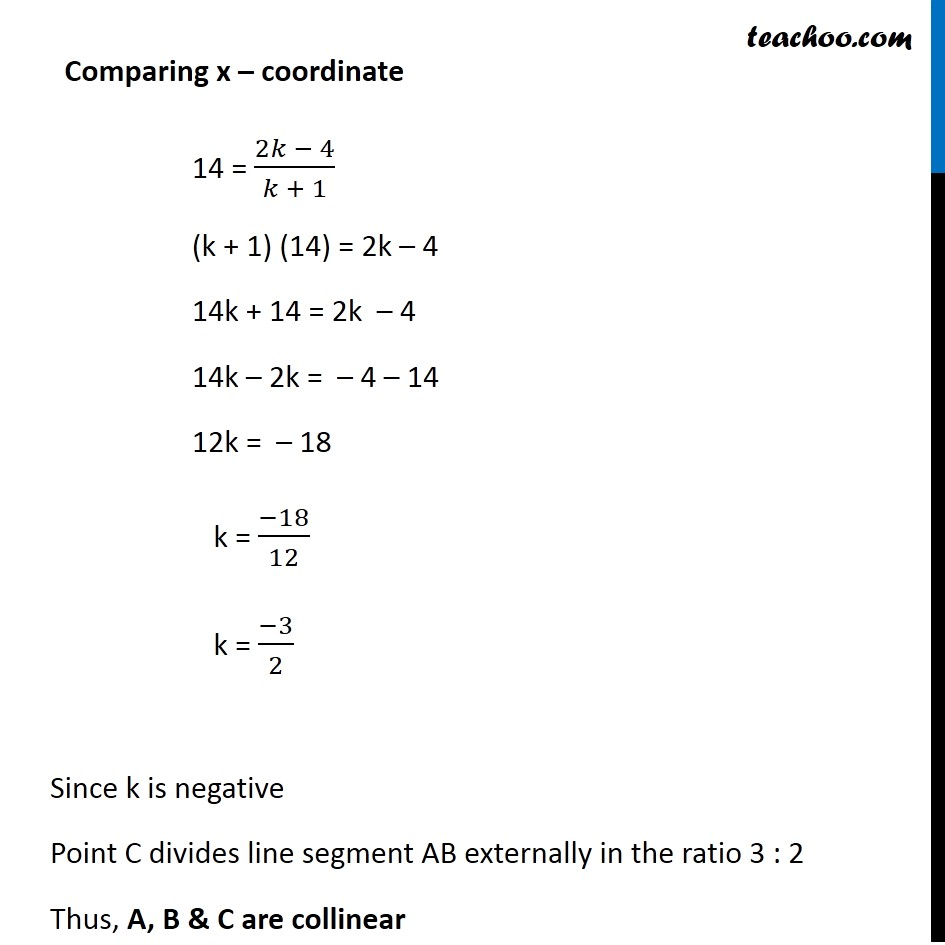Section - Collinear

Chapter 12 Class 11 - Intro to Three Dimensional Geometry
Concept wiseIntroducing your new favourite teacher - Teachoo Black, at only ₹83 per month

### Transcript

Example 8 Using section formula, prove that the three points (– 4, 6, 10), (2, 4, 6) and (14, 0, –2) are collinear. Let points be A (– 4, 6, 10) , B (2, 4, 6) , C (14, 0, – 2) Point A, B, & C are collinear if point C divides AB in some ratio externally & internally We know that Co-ordinate of point P(x, y ,z) that divides line segment joining (x1, y1, z1) & (x2, y2, z2) in ration m : n is (x, y ,z) = ((mx2 + nx1)/(m + n),(my2 + ny1)/(m + n), (〖𝑚𝑧〗_2 + 〖𝑛𝑧〗_1)/(𝑚 + 𝑛)) Here, let point C(14, 0, – 2) divide A(– 4, 6, 10) , B(2, 4, 6) in the ratio k : 1 Here, x1 = – 4, y1 = 6, z1 = 10 x2 = 2, y2 = 4, z2 = 6 & m = k , n = 1 Putting values (14, 0, – 2) = ((𝑘(2) + 1(−4))/(𝑘 + 1),(𝑘(4) + 1 (6))/(𝑘 + 1),(𝑘 (6) + 1 (10))/(𝑘 +1)) (14, 0, – 2) = ((2𝑘 − 4 )/( 𝑘 + 1),(4𝑘 + 6)/(k +1),(6𝑘 + 10)/( k + 1)) Comparing x – coordinate Comparing x – coordinate 14 = (2𝑘 − 4)/(𝑘 + 1) (k + 1) (14) = 2k – 4 14k + 14 = 2k – 4 14k – 2k = – 4 – 14 12k = – 18 k = (−18)/12 k = (−3)/2 Since k is negative Point C divides line segment AB externally in the ratio 3 : 2 Thus, A, B & C are collinear